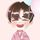cancel
Showing results for
Did you mean:New Member

## Power Query - How to move data to new row and new column?

I have data that I'd like to move but I can't figure out how. I can move to a new column no problem, but I can't get it to move to different rows as shown. Ideas appreciated.

thanks1 ACCEPTED SOLUTIONMicrosoft

Here's one way to do it in the query editor.  To see how it works, just create a blank query, open the Advanced Editor and replace the text there with the M code below.

``````let
Source = Table.FromRows(Json.Document(Binary.Decompress(Binary.FromText("i45WMlTSUQrJyMxLVwCxDA2AhIVSrE60khEuCWNMCUuwBMIokF5DQyxGgSWMsBgFljCGGBULAA==", BinaryEncoding.Base64), Compression.Deflate)), let _t = ((type nullable text) meta [Serialized.Text = true]) in type table [#"Part Number" = _t, Part = _t, Depth = _t, Height = _t]),
#"Changed Type" = Table.TransformColumnTypes(Source,{{"Part Number", Int64.Type}, {"Part", type text}, {"Depth", Int64.Type}, {"Height", Int64.Type}}),
#"Unpivoted Other Columns" = Table.UnpivotOtherColumns(#"Changed Type", {"Part Number"}, "Attribute", "Value"),
#"Added Custom" = Table.AddColumn(#"Unpivoted Other Columns", "Custom", each if [Attribute] = "Part" then [Value] else null),
#"Filtered Rows" = Table.SelectRows(#"Filled Down", each ([Value] <> "Thing 1" and [Value] <> "Thing 2")),
[Custom] = "Thing 1" and [Attribute] = "Depth" then "Depth" else
if [Custom] = "Thing 1" and [Attribute] = "Height" then "Height" else
if [Custom] = "Thing 2" and [Attribute] = "Depth" then "Width" else
if [Custom] = "Thing 2" and [Attribute] = "Height" then "Remove" else null),
#"Filtered Rows1" = Table.SelectRows(#"Added Custom1", each ([Dimension] <> "Remove")),
#"Removed Other Columns" = Table.SelectColumns(#"Filtered Rows1",{"Part Number", "Dimension", "Value"}),
#"Pivoted Column" = Table.Pivot(#"Removed Other Columns", List.Distinct(#"Removed Other Columns"[Dimension]), "Dimension", "Value")
in
#"Pivoted Column"``````

Pat

Did I answer your question? Mark my post as a solution! Kudos are also appreciated!

3 REPLIES 3Community Support

Hi @Scotsman ,

``Rank = CONVERT( RIGHT([Part],1),INTEGER) ``
``Width = LOOKUPVALUE('Table'[Depth],[Part Number],[Part Number],[Rank],[Rank]+1, BLANK())``
``Height2 = IF([Rank]=1,[Height],BLANK()) ``

Output:Best Regards,
Eyelyn Qin
If this post helps, then please consider Accept it as the solution to help the other members find it more quickly.Microsoft

Here's one way to do it in the query editor.  To see how it works, just create a blank query, open the Advanced Editor and replace the text there with the M code below.

``````let
Source = Table.FromRows(Json.Document(Binary.Decompress(Binary.FromText("i45WMlTSUQrJyMxLVwCxDA2AhIVSrE60khEuCWNMCUuwBMIokF5DQyxGgSWMsBgFljCGGBULAA==", BinaryEncoding.Base64), Compression.Deflate)), let _t = ((type nullable text) meta [Serialized.Text = true]) in type table [#"Part Number" = _t, Part = _t, Depth = _t, Height = _t]),
#"Changed Type" = Table.TransformColumnTypes(Source,{{"Part Number", Int64.Type}, {"Part", type text}, {"Depth", Int64.Type}, {"Height", Int64.Type}}),
#"Unpivoted Other Columns" = Table.UnpivotOtherColumns(#"Changed Type", {"Part Number"}, "Attribute", "Value"),
#"Added Custom" = Table.AddColumn(#"Unpivoted Other Columns", "Custom", each if [Attribute] = "Part" then [Value] else null),
#"Filtered Rows" = Table.SelectRows(#"Filled Down", each ([Value] <> "Thing 1" and [Value] <> "Thing 2")),
[Custom] = "Thing 1" and [Attribute] = "Depth" then "Depth" else
if [Custom] = "Thing 1" and [Attribute] = "Height" then "Height" else
if [Custom] = "Thing 2" and [Attribute] = "Depth" then "Width" else
if [Custom] = "Thing 2" and [Attribute] = "Height" then "Remove" else null),
#"Filtered Rows1" = Table.SelectRows(#"Added Custom1", each ([Dimension] <> "Remove")),
#"Removed Other Columns" = Table.SelectColumns(#"Filtered Rows1",{"Part Number", "Dimension", "Value"}),
#"Pivoted Column" = Table.Pivot(#"Removed Other Columns", List.Distinct(#"Removed Other Columns"[Dimension]), "Dimension", "Value")
in
#"Pivoted Column"``````

Pat

Did I answer your question? Mark my post as a solution! Kudos are also appreciated!New Member

Thanks for the response - it will take me some time to apply this bc I am noob. I understand the filtering and the formula, but still trying to get my head around pivoting. I'll get it though - thanks so much.Announcements#### Exclusive opportunity for Women!

Join us for a free, hands-on Microsoft workshop led by women trainers for women where you will learn how to build a Dashboard in a Day!#### Power Platform Conference-Power BI and Fabric Sessions

Join us Oct 1 - 6 in Las Vegas for the Microsoft Power Platform Conference.Top Solution Authors
Top Kudoed Authors
Users online (2,393)